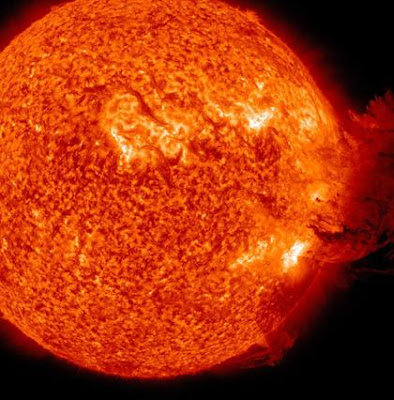## Friday, June 10, 2011

### Accounting for Eruptive Solar Flares (2)In the previous instalment, we found for a hypothetical two-loop model, the relative helicity at outset:

£ARCH BC, AA’ = 5/ 24

It is tempting to attribute this to a relative measure for the mutual inductance of the respective loops, which could fit in with the overall time development. However, as DeMoulin et al (op. cit., 2006) have shown, it is incorrect to render such an association as a generalization. In order for it to hold true that M12 = M21 = M (where M12 is the mutual inductance of loop 1 relative to 2, and M21 of loop 2 relative to 1) we must have both currents in both coils (for N1 and N2) at effectively the same distance r from the center of the loop. Thus, for most 2-loop interacting models this will not be so.

However, for the sake of ease in computation and to minimize complexity, let's assume for Fig 2 (model loops shown in previous instalment):

(B+C-)/ 2 ~ (A+A-)/2 = r

And it is feasible to at least obtain a “guesstimate” of physical quantities.

If the self-inductance of the system is therefore defined:

Lij = (alph_i+ alph_j) (Mij) / u_o

where the alph_i,j are the respective loop force-free scale factors (viz. alph = u_o J_z / B_z where J_z is the vertical current density and B_z the vertical magnetic induction) and ought not be confused with (a1 + a2) the interior angles from the geometry, and u_o is the magnetic permeability of free space (4π x 10^-7 H/m). Then the mutual inductance for the 2-loop system is:

Mij ~ 5/24 = u_o Lij /(alph_i+ alph_j)

For the simplified model (based on Fig. 2 - with simplifying assumptions above) we find that for a self-inductance: Lij = 0.025H (henries).

Also:

(alph_i+ alph_ j) = 1.5 x 10^ –7 (/m)

which more than satisfies the condition for attainment of the critical twist: φ_crit = 2.5π, with the dipole (BC) subject to the MHD kink instability and likely release of magnetic energy. This ought to also have a magnitude great enough to induce an eruptive prominence or CME.

It should also be evident that Poisson behavior can be incepted by variations in the rate of island fusion, and in turn variation in the magnitude of Lij. ("Poisson behavior" refers to flare incidence conforming to Poisson statistics, e.g. of the form: P(N) = exp (- f) f^N / N!, where f is the mean rate of flare production, that is, the mean number of flares per spot group per day.)

For example, if the rate of island fusion allows Lij ~ 50 micro-henries (50mH), then:

alph_i = alph_j = 1.5 x 10^ –10 ( m^–1)

and the force-free scale factors (alph_i,j) are more than two orders of magnitude below what is required for kink instability. In a Poisson statistical formulation, this will present a “no flare” day provided the condition lasts during all observations windows. It is evident from this that a necessary condition for a flare trigger to be in place entails that the rate of fusion d(ff) /dt = Lij > 0.025H.

It is proposed that for the eruptive flare that occurred earlier this week, such a condition was met, though it is expected that the relative magnetic helicity subsumed the mutual inductance in destabilizing the loop system.

Say then for the times t0 and t2, one had two self-inductances, L0, L2 with L0= 4.3H and L2 = 5.6H (reasonable magnitudes for the angular increments assigned).

Then the rate of change in self-inductance:

dL/dt ~ [5.6 H – 4.3H]/ 15s = 0.087 H/s

If the necessary voltage for a flare is defined:

V(t) = I dL(t)/dt

Then the current (I) needed and also V(t) can be found if adequate observational datums are present.

For example, one can obtain the current in the 2-loop system from (cf. D.B. Melrose, Conservation of Both Current and Helicity in a Quadripolar Model for Solar Flares, Solar Phys., 2004)

I_o = [alph_I φ_i]/ u_o

where alph_I is an average of the force-free parameters alph_i,j that is obtained over the loop cross sections, and the magnetic flux of the ith loop (φ_i) is computed from the equipartition value of the magnetic field (B) using:

φ_i = BA

where B is the measured magnetic induction (say from a vector magnetograph) and A is the estimated cross-sectional area.

With alph_I and φ_i known then I_o is found, and thence, V(t) estimated.

When the full data for the recent eruptive solar flare becomes available, I will show how this can be worked out!